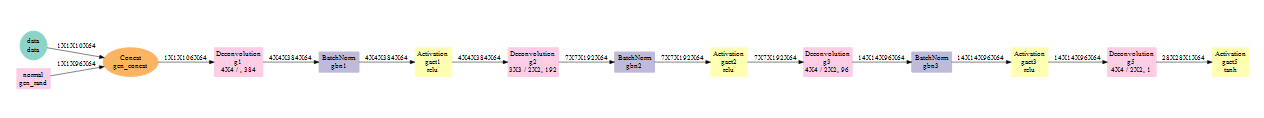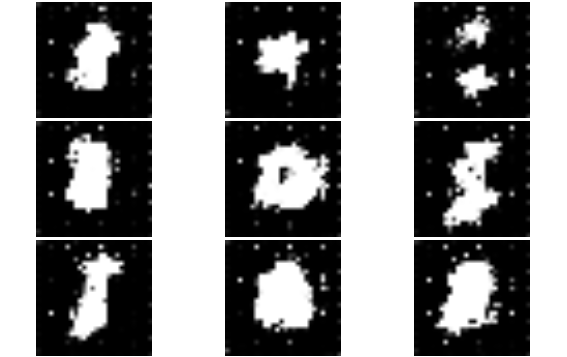Want to share your content on R-bloggers? click here if you have a blog, or here if you don't.

This tutorial shows how to build and train a Conditional Generative Adversial Network (CGAN) on MNIST images.

### How GAN works

A Generative Adversial Model simultaneously trains two models: a generator that learns to output fake samples from an unknown distribution and a discriminator that learns to distinguish fake from real samples.

The CGAN is a conditional variation of the GAN where the generator is instructed to generate a real sample having specific characteristics rather than a generic sample from full distribution. Such condition could be the label associated with an image like in this tutorial or a more detailed tag as shown in the example below:Image credit: Scott Reed

### Initial setup

The following packages are needed to run the tutorial:

``````require("imager")
require("dplyr")
require("mxnet")
``````

The full demo is comprised of the two following scripts available on GitHub:

• `CGAN_mnist_setup.R`: prepare data and define the model structure
• `CGAN_train.R`: execute the training

### Data preperation

The MNIST dataset is available on Kaggle. Once `train.csv` is downloaded into the `data/` folder, we can import into R.

``````train <- read_csv('data/train.csv')
train <- data.matrix(train)

train_data <- train[,-1]
train_data <- t(train_data/255*2-1)
train_label <- as.integer(train[,1])

dim(train_data) <- c(28, 28, 1, ncol(train_data))
``````

Custom iterators are defined in `iterators.R` and imported by `CGAN_mnist_setup.R`.

### Generator

The generator is a network that creates novel samples (MNIST images) from 2 inputs:

• Noise vector
• Labels defining the object condition (which digit to produce)

The noise vector provides the building blocks to the Generator model, which will learns how to structure that noise into a sample. The `mx.symbol.Deconvolution` operator is used to upsample the initial input from a 1×1 shape up to a 28×28 image.

The information on the label for which to generate a fake sample is provided by a one-hot encoding of the label indices that is appended to the random noise. For MNIST, the 0-9 indices are therefore converted into a binary vector of length 10. More complex applications would require embeddings rather than simple one-hot to encode the condition.### Discriminator

The discriminator attempts to distinguish between fake samples produced by the generator and real ones sampled from MNIST training data.

In a conditional GAN, the labels associated with the samples are also provided to the Discriminator. In this demo, this information is again provided as a hot-hot encoding of the label that is broadcast to match the image dimensions (10 -> 28x28x10).### Training logic

The training process of the discriminator is most obvious: the loss is simple a binary TRUE/FALSE response and that loss is propagated back into the CNN network. It can therefore be understood as a simple binary classification problem.

``````### Train loop on fake
mx.exec.update.arg.arrays(exec_D, arg.arrays =
list(data=D_data_fake, digit=D_digit_fake, label=mx.nd.array(rep(0, batch_size))),
match.name=TRUE)
mx.exec.forward(exec_D, is.train=T)
mx.exec.backward(exec_D)
mx.exec.update.arg.arrays(exec_D, update_args_D, skip.null=TRUE)

### Train loop on real
mx.exec.update.arg.arrays(exec_D, arg.arrays =
list(data=D_data_real, digit=D_digit_real, label=mx.nd.array(rep(1, batch_size))),
match.name=TRUE)
mx.exec.forward(exec_D, is.train=T)
mx.exec.backward(exec_D)
mx.exec.update.arg.arrays(exec_D, update_args_D, skip.null=TRUE)
``````

The generator loss comes from the backpropagation of the the discriminator loss into its generated output. By faking the generator labels to be real samples into the discriminator, the discriminator back-propagated loss provides the generator with the information on how to best adapt its parameters to trick the discriminator into believing the fake samples are real.

This requires to backpropagate the gradients up to the input data of the discriminator (whereas this input gradient is typically ignored in vanilla feedforward network).

``````### Update Generator weights - use a seperate executor for writing data gradients
exec_D_back <- mxnet:::mx.symbol.bind(symbol = D_sym,
arg.arrays = exec_D\$arg.arrays,
aux.arrays = exec_D\$aux.arrays, grad.reqs = rep("write", length(exec_D\$arg.arrays)),
ctx = devices)

mx.exec.update.arg.arrays(exec_D_back, arg.arrays =
list(data=D_data_fake, digit=D_digit_fake, label=mx.nd.array(rep(1, batch_size))),
match.name=TRUE)
mx.exec.forward(exec_D_back, is.train=T)
mx.exec.backward(exec_D_back)

mx.exec.update.arg.arrays(exec_G, update_args_G, skip.null=TRUE)
``````

The above training steps are executed in the `CGAN_train.R` script.

### Monitor the training

During training, the imager package facilitates the visual quality assessment of the fake samples.

``````if (iteration==1 | iteration %% 100==0){
par(mfrow=c(3,3), mar=c(0.1,0.1,0.1,0.1))
for (i in 1:9) {
img <- as.array(exec_G\$ref.outputs\$G_sym_output)[,,,i]
plot(as.cimg(img), axes=F)
}
}
``````

Below are samples obtained at different stage of the training.

Starting from noise:Slowly getting it – iteration 200:Generate specified digit images on demand – iteration 2400:### Inference

Once the model is trained, synthetic images of the desired digit can be produced by feeding the generator with fixed labels rather than the randomly generated ones used during the training.

Here we will generate fake “9”:

``````digit <- mx.nd.array(rep(9, times=batch_size))
data <- mx.nd.one.hot(indices = digit, depth = 10)
data <- mx.nd.reshape(data = data, shape = c(1,1,-1, batch_size))

exec_G <- mx.simple.bind(symbol = G_sym, data=data_shape_G, ctx = devices, grad.req = "null")
mx.exec.update.arg.arrays(exec_G, G_arg_params, match.name=TRUE)
mx.exec.update.arg.arrays(exec_G, list(data=data), match.name=TRUE)
mx.exec.update.aux.arrays(exec_G, G_aux_params, match.name=TRUE)

mx.exec.forward(exec_G, is.train=F)
``````Further details of the CGAN methodology can be found in the paper Generative Adversarial Text to Image Synthesis.